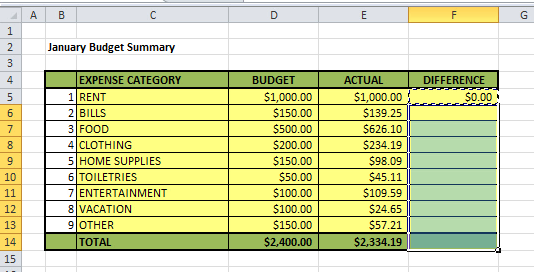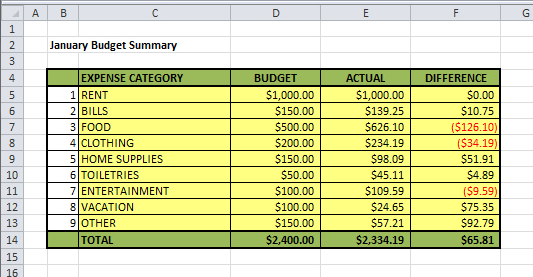# Paste Special Formulas

This is the second part of my Paste Special Series, where I discuss the three most frequently used types of paste special – paste special formats, paste special formulas, and paste special values.

In my last post I talked about paste special formats, and in today’s post I’ll discuss paste special formulas. I use paste special formulas all the time. When you’re creating giant spreadsheets with thousands of rows and highly complex formulas, paste special formulas is a godsend.

## Paste Special Formulas

With paste special formulas, you’re pasting only the formula of something you previously copied or cut. You’re not pasting the formatting of the copied/cut cell. In other words, when you paste special formulas, the format that was already there will stay there.

Another very important thing to note is that when you paste special formulas, Excel will adjust your formula based on the location of your paste. I’ll explain what I mean by this later when we go through our example.

Let’s use the same simple budget data from the last post. Download my simple budget data for the paste special formulas example.

Our simple budget looked like this when we finished creating the difference column.Now let’s add formulas to the difference column. To do this, we’re going to type in the difference formula in the first cell, and copy the formula down to the rest of the cells using paste special formulas.

First enter in the difference formula in cell F5.Then copy cell F5 either by right-clicking, or pressing CTRL+C.

Now select the F6:F14 since this is where you will want to paste your formulas. Then go to the Paste Special Menu by right-clicking and selecting Paste Special, or by pressing ALT, H, V, S.This time when you see the Paste Special menu, select Formulas, and click OK.Notice the formula has now been copied to the other cells.Notice also that the original formatting of the difference column stayed intact. If we had pasted regular (instead of using paste special formulas), we would mess up the nice formatting that was already in there. This is what it would have looked like.Notice how the formatting in F14 looks the same as the cells above it? That’s because you not only pasted the formula of cell F5, but you also pasted its formatting. You don’t have this problem when you do paste special formulas.

The other important thing to point out is what when you pasted special formulas, your formulas did not get pasted exactly as is. Look carefully at the formula in F6.What’s different about this formula vs the one in you entered F5? If you said F6 is taking the difference of D6-E6, whereas F5 is taking the difference of D5-E5, you would be correct. When you pasted special formulas into F6, Excel was smart enough to know that you wanted the formula look at the BUDGET and ACTUAL from row 6 instead of row 5. The same goes for row 7, and 8, and so on.

How did Excel know that? The answer has to do with relative vs absolute cell references, which is a topic for another dayFor now, just know that because there was no “\$” in the formula you typed in F5, your formulas were automatically adjusted to use the corresponding rows when you copied and pasted them down.

I hope you can see how paste special formulas can save you a ton of time when you have to enter the same formula over and over again!

Next week, I’ll talk about the last of my Paste Special Series – paste special values.